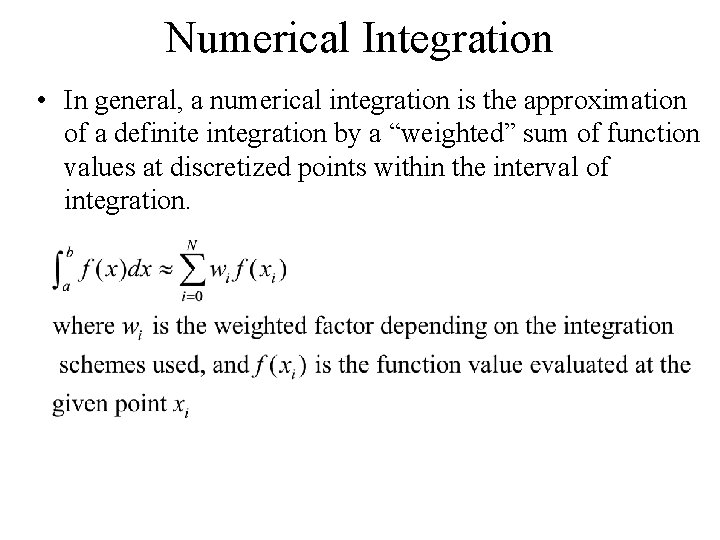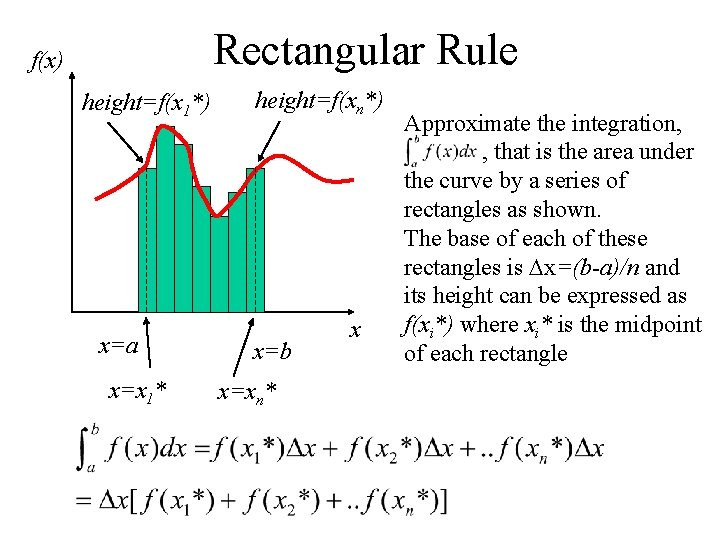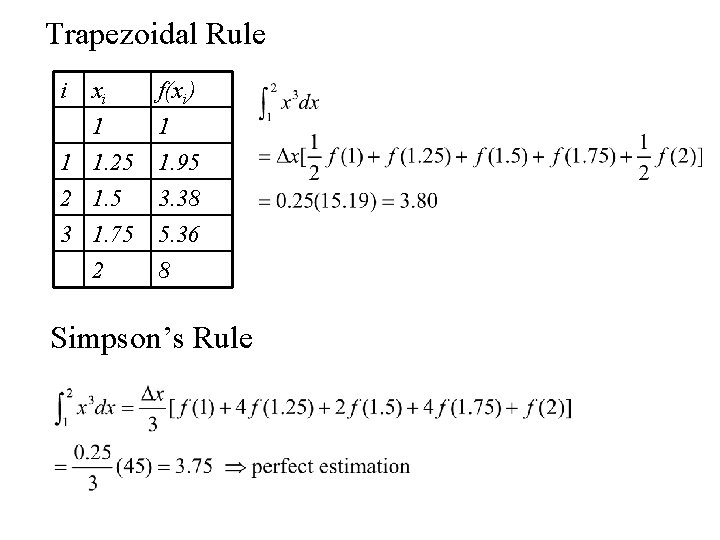# Numerical Integration In general a numerical integration is

• Slides: 6Numerical Integration • In general, a numerical integration is the approximation of a definite integration by a “weighted” sum of function values at discretized points within the interval of integration.Rectangular Rule f(x) height=f(x 1*) x=a x=x 1* height=f(xn*) x=b x=xn* x Approximate the integration, , that is the area under the curve by a series of rectangles as shown. The base of each of these rectangles is Dx=(b-a)/n and its height can be expressed as f(xi*) where xi* is the midpoint of each rectangleTrapezoidal Rule f(x) x=a x=x 1 x=b x=xn-1 x The rectangular rule can be made more accurate by using trapezoids to replace the rectangles as shown. A linear approximation of the function locally sometimes work much better than using the averaged value like the rectangular rule does.Simpson’s Rule Still, the more accurate integration formula can be achieved by approximating the local curve by a higher order function, such as a quadratic polynomial. This leads to the Simpson’s rule and the formula is given as: It is to be noted that the total number of subdivisions has to be an even number in order for the Simpson’s formula to work properly.Examples i 1 2 3 x i* 1. 125 1. 375 1. 625 f(xi*) 1. 42 2. 60 4. 29 4 1. 875 6. 59Trapezoidal Rule i xi 1 1 1. 25 2 1. 5 f(xi) 1 1. 95 3. 38 3 1. 75 2 5. 36 8 Simpson’s Rule